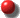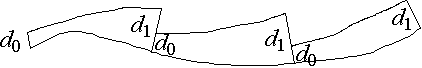# Strange Attractors

## 10/10/00 Lecture #6 in Physics 505

###Comments on Homework #4 (Lorenz Attractor)

• Everyone did a good job
• To get smooth graphs, make h smaller or connect the dots

###Review (last week) - Lyapunov Exponents

• Lyapunov Exponents are a dynamical measure of chaos
• There are as many exponents as the system has dimensions
• dV/dt / V = l1 + l2 + l3 + ...
• = <log |det J|> for maps
• = <trace J> = <fx + gy + hz + ...> for flows
• Where J is the Jacobian matrix
• Sl must be negative for an attractor
• Sl must be zero for a conservative (Hamiltonian) system
• For chaos we require l1 > 0  (at least one positive LE)
• For 1-D Maps, l = <log |df/dX|>
• 2-D example, Hénon map:
• Xn+1 = 1 - CXn2 + BYn   [= f(X, Y)]
• Yn+1 = Xn   [= g(X, Y)]
• Usual parameters for chaos: B = 0.3, C = 1.4
• l1 + l2 = <log |fxgy - fygx|> = log |-B| = -1.204 (base-e)
• Numerical calculation gives l1 = 0.419 (base-e)
• Hence l2 = -1.204 - 0.419 = -1.623 (base-e)
• Fixed points at x* = y* = -1.1313445 and x* = y* = 0.63133545
• General character of Lyapunov exponents in flows:
• (-, -, -, -, ...)  fixed point  (0-D)
• (0, -, -, -, ...)  limit cycle  (1-D)
• (0,0, -, -, ...)  2-torus  (2-D)
• (0, 0, 0, -, ...)  3-torus, etc.  (3-D, etc.)
• (+, 0, -, -, ...)  strange (chaotic)  (2+-D)
• (+, +, 0, -, ...)  hyperchaos, etc.  (3+-D)
• Numerical Calculation of Largest Lyapunov Exponent
• Iterate until the orbit is on the attractor
• Select (almost any) nearby point (separated by d0)
• Advance both orbits one iteration and calculate new separation d1
• Evaluate log |d1/d0| in any convenient base
• Readjust one orbit so its separation is d0 in same direction as d1
• Repeat steps 4-6 many times and calculate average of step 5
• The largest Lyapunov exponent is l1 = <log |d1/d0|>
• If map approximates an ODE, then l1 = <log |d1/d0|> / h
• A positive value of l1 indicates chaos

•• Kaplan-Yorke (Lyapunov) Dimension
• Attractor dimension is a geometrical measure of complexity
• Random noise is infinite dimensional (infinitely complex)
• How do we calculate the dimension of an attractor?  (many ways)
• Suppose system has dimension N  (hence N Lyapunov exponents)
• Suppose the first D of these sum to zero
• Then the attractor would have dimension D
• (in D dimensions there would be neither expansion nor contraction)
• In general, find the largest D for which l1 + l2 + ... + lD > 0
• (The integer D is sometimes called the topological dimension)
• The attractor dimension would be between D and D + 1
• However, we can do better by interpolating:
• DKY = D + (l1 + l2 + ... + lD) / |lD+1|
• The Kaplan-Yorke conjecture is that DKY agrees with other methods
• 2-D Map Example: Hénon map  (B = 0.3, C = 1.4)
• l1 = 0.419 and l2 = -1.623
• D = 1 and DKY = 1 + l1 / |l2| = 1 + 0.419 / 1.623 = 1.258
• Agrees with intuition and other calculations
• 3-D Flow Example:  Lorenz Attractor  (p = 10, r = 28,  b = 8/3)
• Numerical calculation gives l1 = 0.906
• Since it is a flow, l2 = 0
• l1 + l2 + l3 = <fx + gy + hz> = -p - 1 - b = -13.667
• Therefore, l3 = -14.572
• D = 2 and DKY = 2 + l1 / |l3| = 2 + 0.906 / 14.572 = 2.062
• Chaotic flows always have DKY > 2
• Chaotic maps always have DKY > 1
• Higher order interpolations are possible
• Precautions
• Be sure orbit is bounded and looks chaotic
• Be sure orbit has adequately sampled the attractor
• Watch for contraction to zero within machine precision
• Test with different initial conditions, step size, etc.
• Supplement with other tests (Poincaré section, Power spectrum, etc.)

###Strange Attractors

J. C. Sprott | Physics 505 Home Page | Previous Lecture | Next Lecture